Question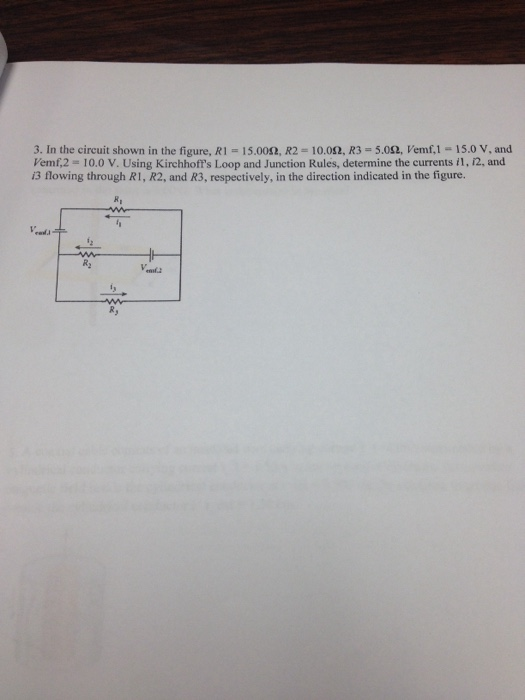Using Kirchoff Voltage & Current Loop,
i1 + i2 = i3 ------1
i2*R2 + i3*R3 = V2
i2*10.0 + i3 * 5.0 = 10.0 -----2
i1*R1 - V1 + i3*r3 = 0
i1*15.0 + i3*5.0 = 15.0 ---------3

Soilving eq 1, 2 & 3
i1 = 0.636 A
i2 = 0.455 A
i3 = 1.09 A

#### Earn Coins

Coins can be redeemed for fabulous gifts.

Similar Homework Help Questions
• ### R1 = 5.79 ?, R2 = 10.3 ?, and R3 = 15.1 ?, Vemf,1 = 12.1 V, and Vemf,2 = 15.9 V. Using...

In the circuit shown in the figure, R1 = 5.79 Ω, R2 = 10.3 Ω, and R3 = 15.1 Ω, Vemf,1 = 12.1 V, and Vemf,2 = 15.9 V. Using Kirchhoff's Loop and JunctionRules, determine the following currents:a) Current i1 flowing through resistor R1 in the direction indicated in the figure.b) Current i2 flowing through resistor R2 in the direction indicated in the figurec) Current i3 flowing through resistor R3 in the direction indicated in the figure.

• ### Assume the resistance values are R1 = 2,200 Ohm, R2 = 1,300 Ohm, R3 = 4,200...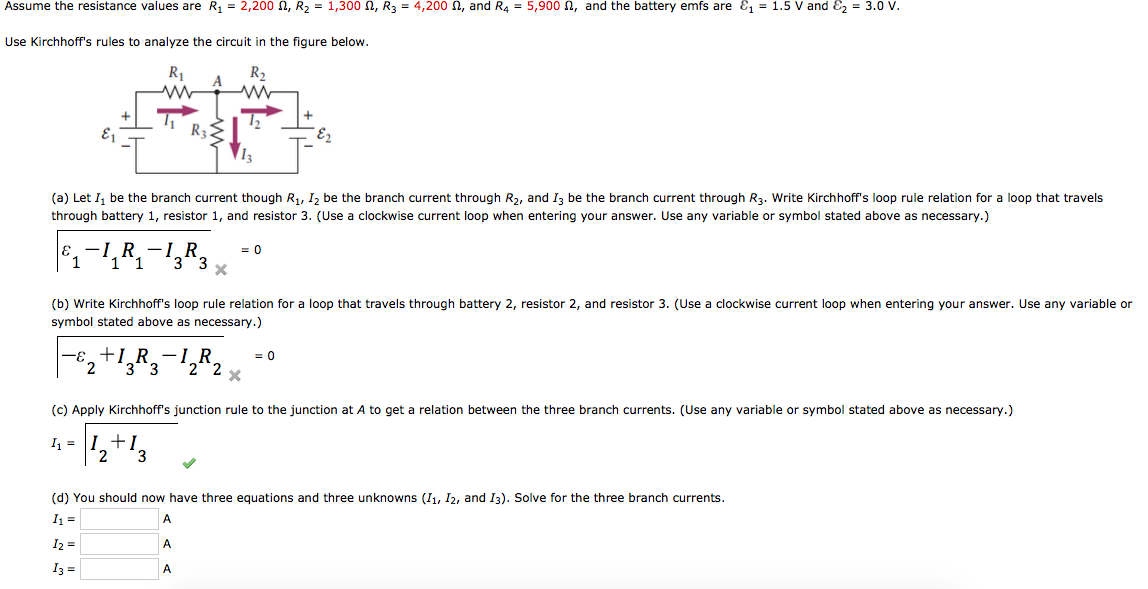Assume the resistance values are R1 = 2,200 Ohm, R2 = 1,300 Ohm, R3 = 4,200 Ohm, and R4 = 5,900 Ohm, and the battery emfs are epsilon1 = 1.5 V and Epsilon2 = 3.0 V. Use Kirchhoff?s rules to analyze the circuit in the figure below. (a) Let I1 be the branch current though R1, I2 be the branch current through R2, and I3 be the branch current through R3. Write Kirchhoff?s loop rule relation for a loop that...

• ### For the above circuit, E1 = 3.57 V, E2 = 5.79 V. r1 = 0.591 Ω, r2 = 0.781 Ω, R1 = 41.3 Ω, R2 = 65...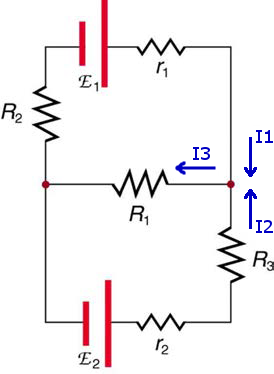For the above circuit, E1 = 3.57 V, E2 = 5.79 V. r1 = 0.591 Ω, r2 = 0.781 Ω, R1 = 41.3 Ω, R2 = 65.1 Ω, R3 = 73.3 Ω. a)Which equation would describe Kirchhoff's Junction Law at the right junction? A I1 - I2 = I3 B  I1 + I2 = I3 C I1 + I3 = I2 D I1 = I2 + I3 b)Which equation would describe Kirchhoff's Loop Law for the lower half-loop? A E2...

• ### For the circuit shown in the figure below,we wish to find the currents I1, I2, and...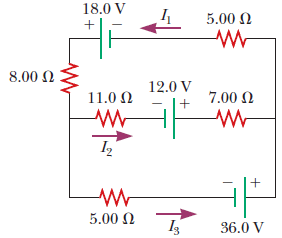For the circuit shown in the figure below,we wish to find the currents I1, I2, and I3. (a) Use Kirchhoff's rules to complete the equation for the upper loop. (Use any variable or symbol stated above as necessary. All currents are given in amperes. Do not enter any units.) 30 V =? (b) Use Kirchhoff's rules to complete the equation for the lower loop. (Use any variable or symbol stated above as necessary. All currents are given in amperes. Do...

• ### Using Kirchhoff's rules, find thecurrents I1, I2, and I3 in the circuit shown where R1...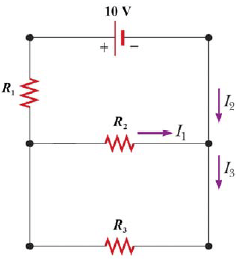Using Kirchhoff's rules, find the currents I1, I2, and I3 in the circuit shown where R1 = 1.2 Ω, R2 = 2.8 Ω, and R3 = 6.3 Ω.

• ### Consider the circuit shown in the figure(Figure 1). Suppose thefour resistors in this circuit have...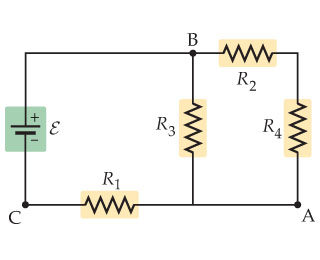Consider the circuit shown in the figure(Figure 1). Suppose the four resistors in this circuit have the values R1 = 13 Ω , R2 = 7.2 Ω , R3 = 6.2 Ω , and R4= 13 Ω , and that the emf of the battery is E = 18 V .Part AFind the current through each resistor using the rules for series and parallel resistors. Express your answers using two significant figures separated by commas.I1,I2,I3,I4=______APart BFind the current through each...

• ### In the circuit shown in the figure below, determine the current in each resistor at the following times. Use r1, r2, an...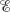In the circuit shown in the figure below, determine the current in each resistor at the following times. Use r1, r2, and r3 for R1, R2, and R3, respectively, and use E for . (a) at the moment the switch is closed I1 =   I2 =   I3 =   (b) a long time after the switch is closed I1 =   I2 =   I3 = After the switch has been closed a long time, and reopened, determine each current at the following...

• ### 1. For the circuit shown, the following is known: R1 = 82, R3 = 1.2, v2...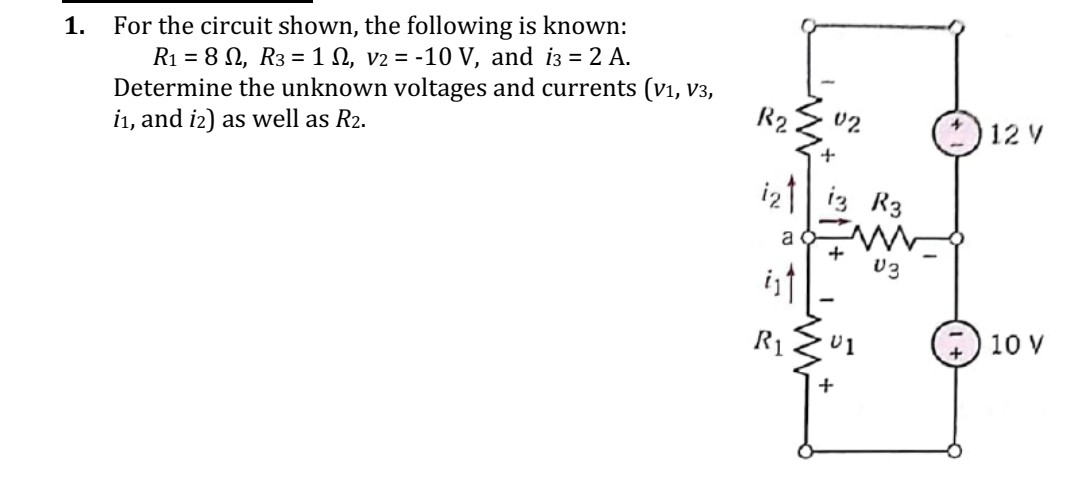1. For the circuit shown, the following is known: R1 = 82, R3 = 1.2, v2 = -10 V, and i3 = 2 A. Determine the unknown voltages and currents (V1, V3, i1, and i2) as well as R2. R2 02 12 V iztiz R3 a 03 R1 U 1 10 V +

• ### The three resistors in the figure are R1 = 25 Ω, R2 = 50 and R3...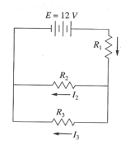The three resistors in the figure are R1 = 25 Ω, R2 = 50 and R3 = 100 Ω. A) what is the total resistance of the circuit? B) what are the currents currents I1, I2 and I3 for a 12V battery? show your work. Ep 12V 三 RE R R; --

• ### Consider the circuit below. If R1=102, R2=212, R3=2012, and Is=10 A, Calculate: 1- The currents i1,...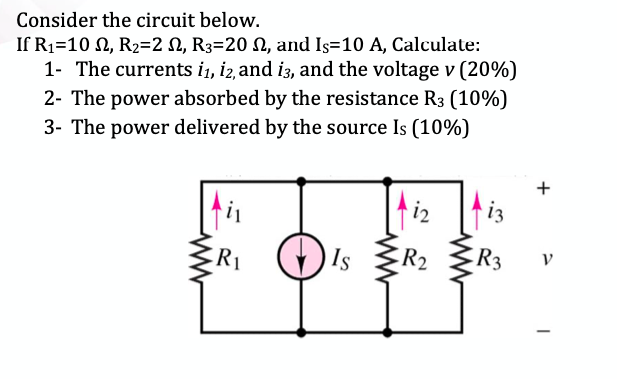Consider the circuit below. If R1=102, R2=212, R3=2012, and Is=10 A, Calculate: 1- The currents i1, 12, and i3, and the voltage v (20%) 2- The power absorbed by the resistance R3 (10%) 3- The power delivered by the source Is (10%) + i2 | 413 w R1 Is R2 R3 V -